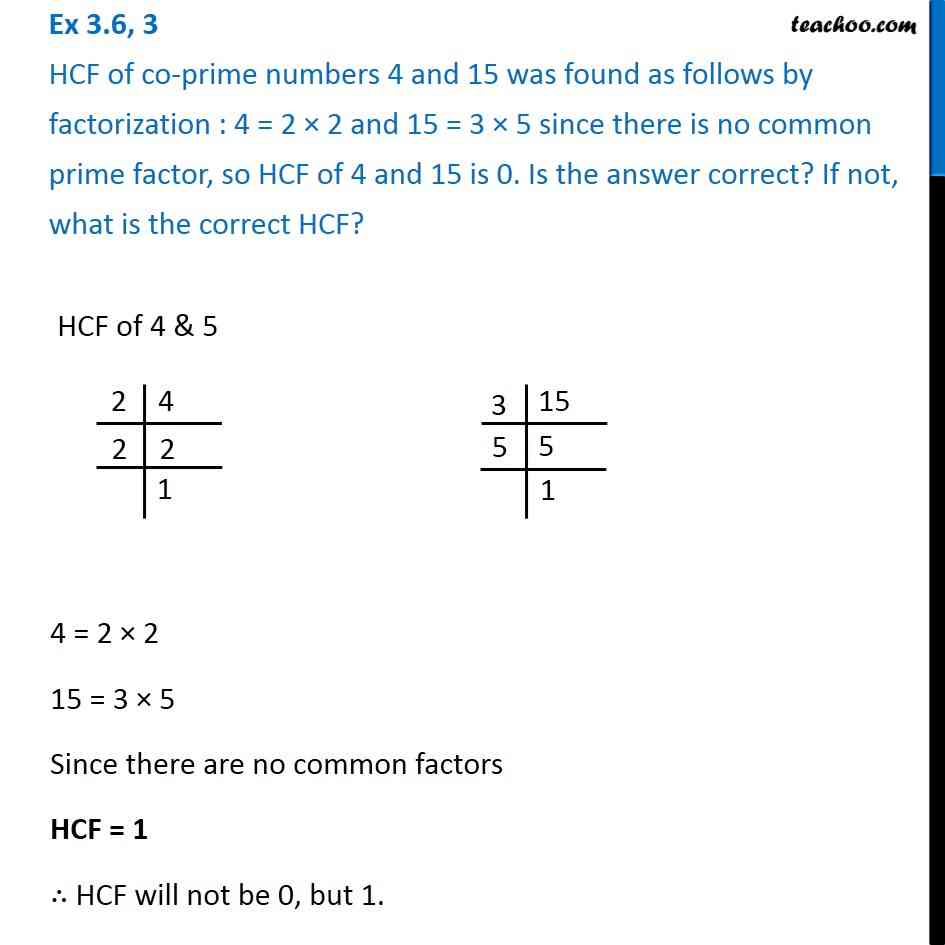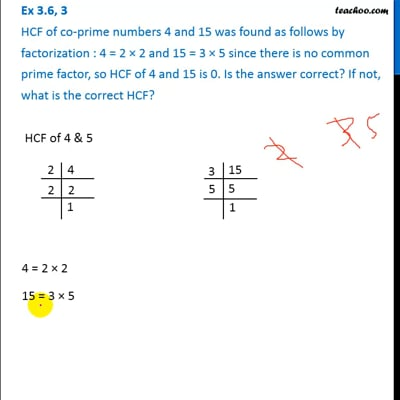Ex 3.6

Chapter 3 Class 6 Playing with Numbers
Serial order wiseThis video is only available for Teachoo black users

Learn in your speed, with individual attention - Teachoo Maths 1-on-1 Class

### Transcript

Ex 3.6, 3 HCF of co-prime numbers 4 and 15 was found as follows by factorization : 4 = 2 × 2 and 15 = 3 × 5 since there is no common prime factor, so HCF of 4 and 15 is 0. Is the answer correct? If not, what is the correct HCF? HCF of 4 & 5 We do prime factorisation of both 4 and 5 Thus, 4 = 2 × 2 15 = 3 × 5 Since there are no prime common factors The only common factor will be 1 So, HCF = 1 ∴ HCF will not be 0, but 1.